## Band Structure p.8

We see here the wavefunctions for various values of V0 and k. The probability densities for the four lowest eigenvectors are presented in "tour" order:

k=(kx,ky)=
(max,0), (2,0), (0,0), (0,2), (0,max)

As you recall for the free lattice, the eigenfunctions are:

exp(iG·r)

so the probability density is uniform. However, our tour of k-space is along high symmetry lines: there is much degeneracy. Any slight perturbation will mix the degenerate wavefunctions to produce linear combinations of the above waves. Linear combinations of the above do not produce uniform probability density. SO here is what is expected for V0 near zero:

### V0=0

``````
Note that the non-degenerate wavefunctions have not been mixed and so have uniform probability densities.

The upper rhs eigenfunctions were seen to have a degeneracy involving the nearest neighbors in the two and ten o'clock positions: what you see displayed is just the plus and minus linear combination of those two exp(iG·r). It will be a nice problem to figure out some of the other linear combinations!

### V0=16

```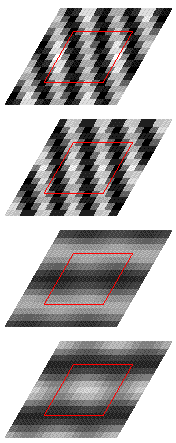```

### V0=64

```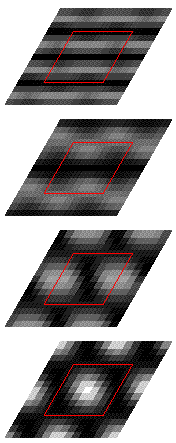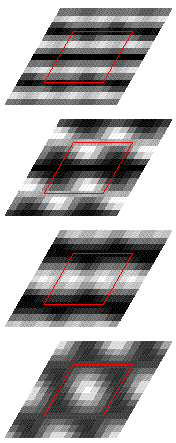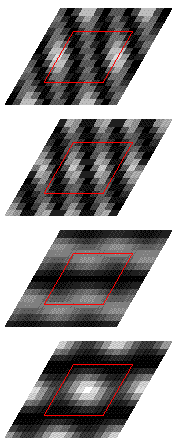```
Notice that the ground state is fairly independent of k. (The gray-scale is constant only within a column of four. Thus even though k=0 ground state looks dimmer than the rest, they are really all similar.

### V0=256

```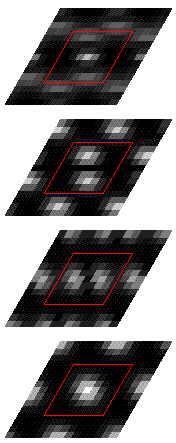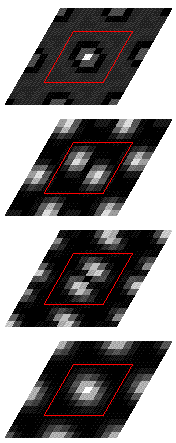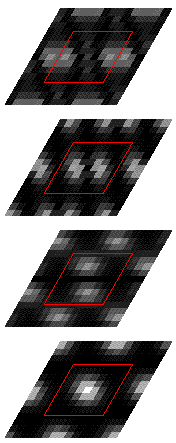```
Here is the summary: we display wavefunctions taken at a fairly random k-space point (not a tour-point; in fact the median of the tour triangle): about k=(0.7,2.4). We display below in succession V0=16,64,256.
```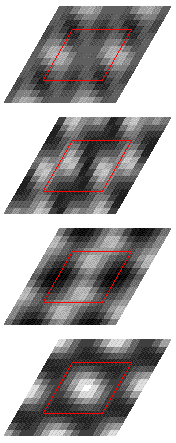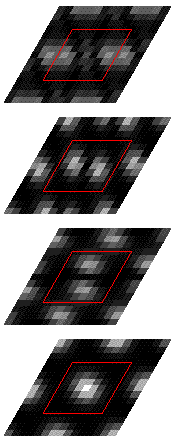```
Notice that we go from a fairly uniform electron density, to something approximating bound states.